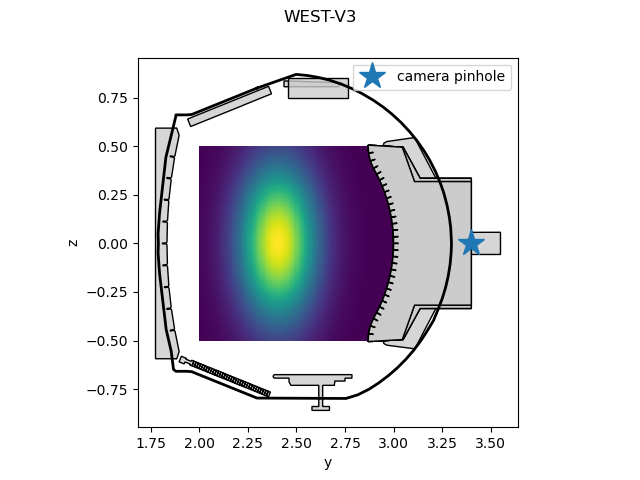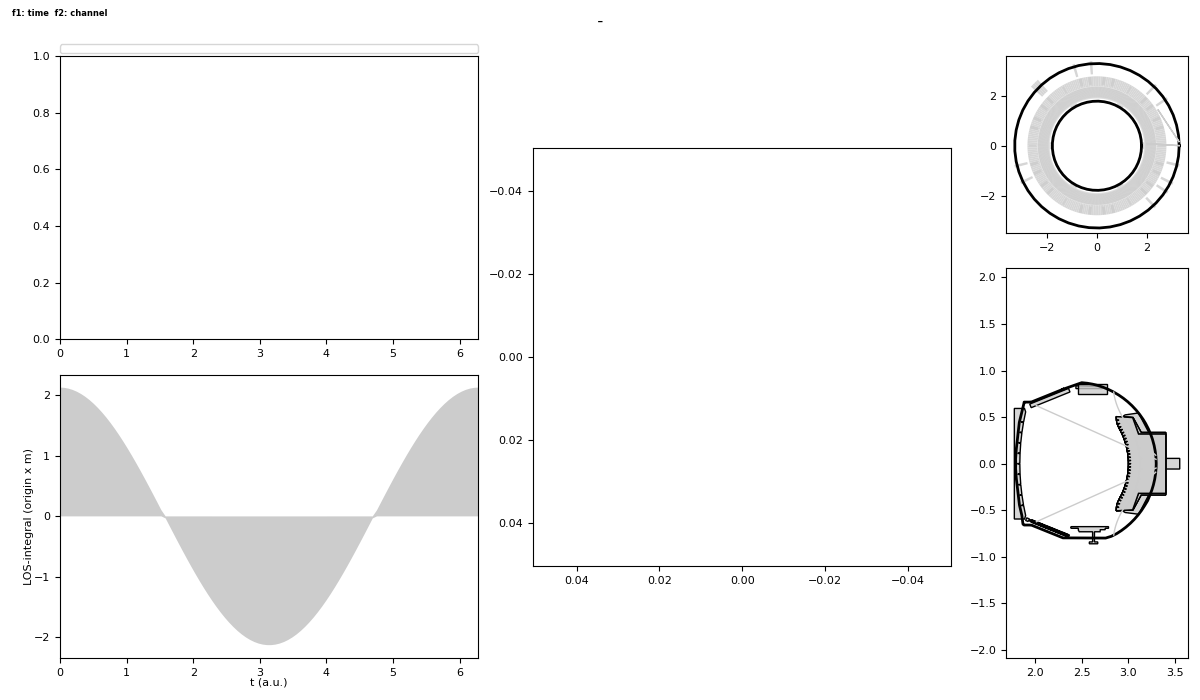# Computing a camera image with custom emissivity¶

This tutorial defines an emissivity that varies in space and computes the signal received by a camera using this emissivity.

We start by loading a built-in tofu configuration and define a 2D camera.

```import matplotlib.pyplot as plt
import numpy as np
import tofu as tf

cam2d = tf.geom.utils.create_CamLOS2D(
config=configB2,
pinhole=[3.4, 0, 0],
sensor_nb=100,
focal=0.1,
sensor_size=0.1,
orientation=[np.pi, np.pi/6, 0],
Name="",
Exp="",
Diag="",
)
```

Out:

```/home/lasofivec/miniconda3/envs/tofu3/lib/python3.8/site-packages/numpy/core/_asarray.py:102: VisibleDeprecationWarning: Creating an ndarray from ragged nested sequences (which is a list-or-tuple of lists-or-tuples-or ndarrays with different lengths or shapes) is deprecated. If you meant to do this, you must specify 'dtype=object' when creating the ndarray.
return array(a, dtype, copy=False, order=order)
```

Now, we define an emissivity function that depends on r and z coordinates. We can plot its profile in the (0, X, Z) plane.

```def emissivity(pts, t=None, vect=None):
"""Custom emissivity as a function of geometry.

:param pts: ndarray of shape (3, n_points) (each column is a xyz coordinate)
:param t: optional, time parameter to add a time dependency to the
emissivity function
:param vect: optional, ndarray of shape (3, n_points), if anisotropic
emissivity, unit direction vectors (X,Y,Z)
:return:
- emissivity -- 2D array holding the emissivity for each point in the
input grid
"""
r, z = np.hypot(pts[0, :], pts[1, :]), pts[2, :]
e = np.exp(-(r - 2.4) ** 2 / 0.2 ** 2 - z ** 2 / 0.4 ** 2)
if t is not None:
e = np.cos(np.atleast_1d(t))[:, None] * e[None, :]
else:
# as stated in documentation of calc_signal, e.ndim must be 2
e = np.reshape(e, (1, -1))
return e

y = np.linspace(2, 3, num=90)
z = np.linspace(-0.5, 0.5, num=100)
Y, Z = np.meshgrid(y, z)
X = np.zeros_like(Y)
pts = np.c_[X.ravel(), Y.ravel(), Z.ravel()].T
emissivity_vals = emissivity(pts)
emissivity_vals = emissivity_vals.reshape(X.shape)

def project_to_2D(xyz):
"""Projection to (0, X, Z) plane."""
return xyz, xyz

fig, ax = plt.subplots()
ax.pcolormesh(Y, Z, emissivity_vals)
ax.set_xlabel('y')
ax.set_ylabel('z')
configB2.plot(lax=ax, proj='cross')
cam_center, = ax.plot(*project_to_2D(cam2d._dgeom['pinhole']), '*', ms=20)
ax.set_aspect("equal")
ax.legend(handles=[cam_center], labels=['camera pinhole'], loc='upper right')
```Out:

```/home/lasofivec/tofu/examples/tutorials/tuto_plot_custom_emissivity.py:72: MatplotlibDeprecationWarning: shading='flat' when X and Y have the same dimensions as C is deprecated since 3.3.  Either specify the corners of the quadrilaterals with X and Y, or pass shading='auto', 'nearest' or 'gouraud', or set rcParams['pcolor.shading'].  This will become an error two minor releases later.
ax.pcolormesh(Y, Z, emissivity_vals)

<matplotlib.legend.Legend object at 0x7f2e2ec0d0d0>
```

Finally, we compute an image using the 2D camera and this emissivity. If we provide a time vector, the field will vary in a cosinusoidal fashion (see above definition) across time.

```time_vector = np.linspace(0, 2 * np.pi, num=100)

sig, units = cam2d.calc_signal(emissivity,
res=0.01,
reflections=False,
minimize="hybrid",
method="sum",
newcalc=True,
plot=False,
ani=False,
t=time_vector)

sig.plot(ntMax=1)
plt.show()
```Out:

```No handles with labels found to put in legend.
/home/lasofivec/tofu/tofu/utils.py:3453: UserWarning: Not interactive backend!:
- backend : agg   (prefer Qt5Agg)
- canvas  : FigureCanvasAgg
warnings.warn(msg)
```

Total running time of the script: ( 0 minutes 2.895 seconds)

Gallery generated by Sphinx-Gallery